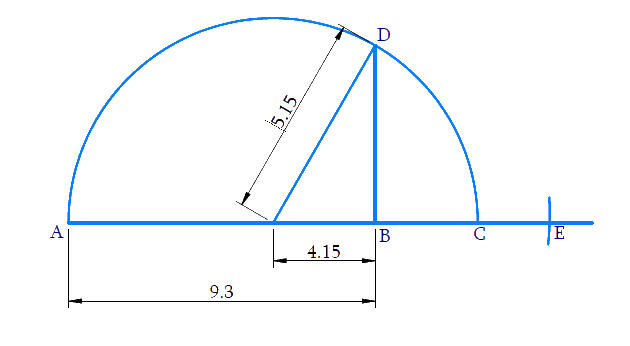# Ex.1.5 Q4 Number System Solution - NCERT Maths Class 9

Go back to  'Ex.1.5'

## Question

Represent \begin{align}\sqrt {9.3} \end{align} on the number line.

Video Solution
Number Systems
Ex 1.5 | Question 4

## Text Solution

Steps:

Draw a line and take $$AB = 9.3$$ units on it.

From $$B$$ measure a distance of $$1$$ unit and mark $$C$$ on the number line. Make the midpoint of $$AC$$ as $$O.$$

With $$‘O’$$ as center and $$OC$$ as radius, draw a semicircle.

At $$B$$, draw a perpendicular to cut the semicircle at $$D$$, with $$B$$ as center and $$BD$$ as radius draw an arc to cut the number line at $$E$$. Taking $$B$$ as the origin the distance $${BE}=\sqrt{9.3}$$ and hence $$E$$ represents $$\sqrt{9.3}$$ .Proof:

\begin{align}AB &=9.3, BC=1 \\ AC &=A B+BC=10.3 \\ OC &=\frac{AC}{2}=\frac{10.3}{2}=5.15 \\ OC&=OD=5.15\\O B &=OC-BC\\&=5.15-1=4.15 \end{align}

In right angled\begin{align}\Delta OBD,\end{align}

\begin{align} {BD}^{2} &={OD}^{2}-{OB}^{2}\\ &=(5.15)^{2}-(4.15)^{2} \\ &=(5.15+4.15)(5.15-4.15)\\&\rm{Using} \quad a^{2}-b^{2}=(a+b)(a-b) \\ &=9.3 \times 1\\ &=9.3 \\ BD &=\sqrt{9.3}=BE \end{align}

Video Solution
Number Systems
Ex 1.5 | Question 4

Learn from the best math teachers and top your exams

• Live one on one classroom and doubt clearing
• Practice worksheets in and after class for conceptual clarity
• Personalized curriculum to keep up with school